# Fluid Mechanics - 1

## 20 Questions MCQ Test Mock Test Series for SSC JE Mechanical Engineering | Fluid Mechanics - 1

Description
Attempt Fluid Mechanics - 1 | 20 questions in 12 minutes | Mock test for SSC preparation | Free important questions MCQ to study Mock Test Series for SSC JE Mechanical Engineering for SSC Exam | Download free PDF with solutions
QUESTION: 1

### What is the specific gravity of a fluid whose specific weight is 7.85 kN/m3?

Solution:

Specific Weight:

$$\gamma = \rho g = 7.85 \Rightarrow \rho = \frac{{7.85 \times {{10}^3}}}{{10}} = 785$$

Specific gravity:

$$s = \frac{\rho }{{{\rho _{water}}}} = \frac{{785}}{{1000}} = 0.8$$

QUESTION: 2

### A pump delivers water at a rate of 0.025 m3/s against a head of 30 m. If the overall efficiency of the pump is 75%, the power required by the pump is:

Solution:

P=ρgQH=1000×9.81×0.025×30=7357.5WP=ρgQH=1000×9.81×0.025×30=7357.5W

Power required: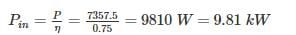QUESTION: 3

### The velocity potential which follow the equation of continuity is ________

Solution:

Velocity Potential function (ϕ) is given as = x2 - y2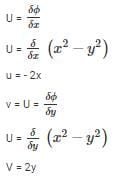For continuity to be satisfied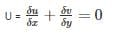-2 + 2 = 0

(Hence satisfied)

QUESTION: 4

When a liquid rotates at a constant angular velocity about a vertical axis as a rigid body, the pressure

Solution:

A fluid is rotating at constant angular velocity ω about the central vertical axis of a cylindrical container. The variation of pressure in the radial direction is given by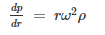It is given that the pressure at the axis of rotation is Pc.

Therefore, the required pressure at any point r is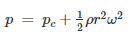QUESTION: 5

Pressure of 200 kPa is equivalent to the head of z metre of liquid having relative density 1.59. The value of z (m) is _____.

Solution:

P = ρgh

h = z

P = ρgz

200 × 103 = 1.59 × 1000 × 9.81 × z

z = 12.82 metres

Where P = Pressure (pascal)

ρ = density of fluid (kg/m3)

g = acceleration due to gravity (m/s2)

h = height of liquid column (metres)

QUESTION: 6

Which of the following is CORRECT about the viscosity of gas?

Solution:

As temperature increases, randomness in molecules also increases. This increase in randomness will result in increase in viscosity as viscosity of gas depends on randomness & collision of gas molecules.

Phenomenon: As a gas is heated, the movement of gas molecules increases and the probability that one gas molecule will collide with another gas molecule increases. In other words, increasing gas temperature causes the gas molecules to collide more often. This increases the gas viscosity because the transfer of momentum between stationary and moving molecules is what causes gas viscosity.

QUESTION: 7

The barometric pressure at the base of a mountain is 750 mm Hg and at the top 600 mm Hg. if the average air density is 1 kg/m3, the height of the mountain (in m) is approximately

Solution:

At base PB = 750 mm of Hg

At top, PA = 600 mm of Hg

∴ Pressure difference = 150 mm of Hg

(ΔP) = hρg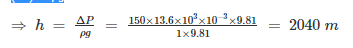⇒ Height of mountain = 2040 m

QUESTION: 8

Runaway speed of a hydraulic turbine is

Solution:

The runaway speed of a water turbine is its speed at full flow, and no shaft load. The turbine will be designed to survive the mechanical forces of this speed.

QUESTION: 9

The centre of pressure on inclined immersed surface remains valid for the centre of pressure on vertical immersed surface, if the angle (θ) at immersed surface with liquid surface is:

Solution:

Centre of Pressure on a Immersed surface from water surface is given by: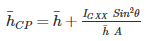For vertical surface θ = 90°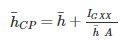So, the formula is valid for θ = 90°

QUESTION: 10

The flow in which the velocity vector is identical in magnitude and direction at every point, for any given instant, is known as

Solution:

The flow is defined as uniform flow when in the flow field the velocity and other hydrodynamic parameters do not change from point to point at any instant of time. For a uniform flow, there will be no spatial distribution of hydrodynamic and other parameters.

When the velocity and other hydrodynamic parameters changes from one point to another the flow is defined as non-uniform.

steady flow is defined as a flow in which the various hydrodynamic parameters and fluid properties at any point do not change with time.

One-dimensional flow is the flow where all the flow parameters may be expressed as functions of time and one space coordinate only. The single space coordinate is usually the distance measured along the centre-line (not necessarily straight) in which the fluid is flowing. Example: the flow in a pipe is considered one - dimensional when variations of pressure and velocity occur along the length of the pipe, but any variation over the cross-section is assumed negligible.

Turbulent fluid motion can be considered as an irregular condition of flow in which various quantities (such as velocity components and pressure) show a random variation with time and space.

QUESTION: 11

Select the correct option for the velocity profile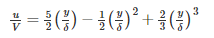Solution:

The separation point S is determined from the condition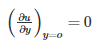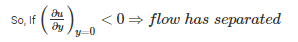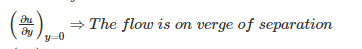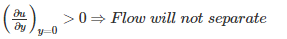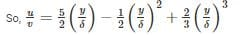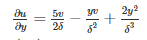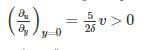⇒ Flow will not separate.

QUESTION: 12

In Kaplan turbine runner, the number of blades is generally of the order _____.

Solution:

Kaplan turbine has adjustable runner blades. Kaplan Turbine has very small number of blades 3 to 8. France Turbine has very large number of blades 16 to 24

QUESTION: 13

ln a two-dimensional flow, where u is the x-component and v is the y-component of velocity the equation of streamline is given by

Solution:

Equation of stream line is given as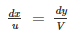⇒ Vdx – udy = 0

QUESTION: 14

Kinematic viscosity of air at 20°C is given to be 1.6 × 10-5 m2/s. Its kinematic viscosity at 70°C will be approximately

Solution:

Dynamic viscosity of gases increase with temp μ∝T−−√μ∝T

Density of gases decreases with increase in temp ρ∝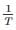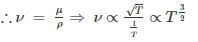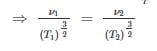T1 = 20 + 273 = 293 K   T2 = 70 + 273 = 343 K
ν1 = 1.6 × 10-5 m2/s       ν2 = ?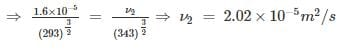QUESTION: 15

The equation gz+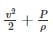constant along a stream line holds true for

Solution:

PdA - (P + dP) dA - ρgdAds sin θ = 0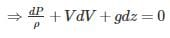⇒dPρ+VdV+gdz=0⇒dPρ+VdV+gdz=0

Assumptions in Bernoulli’s equation:

i) fluid is ideal

iii) flow is continuous

iv) fluid is incompressible

v) flow is non-viscous

vi) flow is irrotational

vii) applicable along a stream line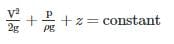where,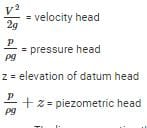• The line representing the sum of all 3 head is known as total energy line or total head line
• Line joining the points of piezometric heads is known as hydraulic grade line or piezometric line.
QUESTION: 16

If for a fluid in motion, the pressure at a point is same in all directions, then the fluid is

Solution:

An ideal fluid is a fluid that has several properties including the fact that it is:

Incompressible - the density is constant with respect to pressure.

Irrotational - the flow is smooth, no turbulence.

Nonviscous - (Inviscid) fluid has no internal friction (η = 0)

QUESTION: 17

A fully developed laminar viscous flow through a circular tube has the ratio of maximum velocity to average velocity as

Solution: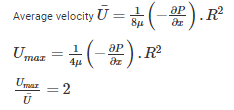QUESTION: 18

The diameter of a soap bubble which has an inside pressure of 2.5 N/mover the atmospheric pressure and a surface tension of 0.0125 N/m is

Solution:

For soap bubble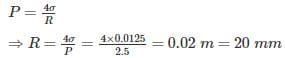∴ Diameter = 40 mm

QUESTION: 19

In Bernoulli’s equation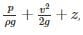each term represents:

Solution:

If the expression is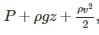then it has units of total energy per unit volume.

The expression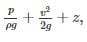, has units of energy per unit weight.

QUESTION: 20

A fluid when acted upon by a shear stress will deform

Solution:

Viscosity is the property of the fluid to resist the rate at which deformation takes place when the fluid is acted upon by shear force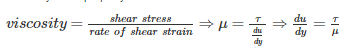A fluid when acted upon by a shear stress will deform when the applied shear stress is greater than the viscous strength of the fluid.Use Code STAYHOME200 and get INR 200 additional OFF Use Coupon Code